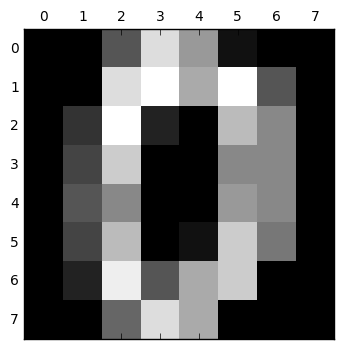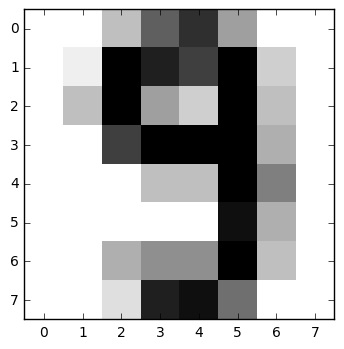# Python SVM Classifier Example¶

(C) 2017-2019 by Damir Cavar

Download: This and various other Jupyter notebooks are available from my GitHub repo.

Version: 1.1, September 2019

This is a tutorial related to the discussion of an SVN classifier in the textbook Machine Learning: The Art and Science of Algorithms that Make Sense of Data by Peter Flach.

This tutorial was developed as part of my course material for the course Machine Learning for Computational Linguistics in the Computational Linguistics Program of the Department of Linguistics at Indiana University.

## SVN Example using¶

The basic idea and storyline for this example was taken from or inspired by the tutorial Simple Support Vector Machine (SVM) example with character recognition.

This tutorial requires Scikit-learn and Matplotlib. These modules come with the default Anaconda Python installation.

To start the tutorial and run the example, we will import pyplot from matplotlib, and datasets and svm from scikit-learn.

In :
import matplotlib.pyplot as plt
from sklearn import datasets
from sklearn import svm


We load the digits data set into memory, refering to it with the variable digits.

In :
digits = datasets.load_digits()


We can output the data set in digits:

In :
print(digits.data)

[[  0.   0.   5. ...,   0.   0.   0.]
[  0.   0.   0. ...,  10.   0.   0.]
[  0.   0.   0. ...,  16.   9.   0.]
...,
[  0.   0.   1. ...,   6.   0.   0.]
[  0.   0.   2. ...,  12.   0.   0.]
[  0.   0.  10. ...,  12.   1.   0.]]


The data contains the actual features. You will find a brief description of the digits dataset on the Scikit-learn website. It contains datapoints with 8x8 images of the digits 0 to 9.

We can print out the image of the digits. In this case we are printing the digit 0:

In :
plt.gray()
plt.matshow(digits.images)
plt.show()

<matplotlib.figure.Figure at 0x1109b2d30>The target vector contains the actual labels of the datapoints.

In :
print(digits.target)

[0 1 2 ..., 8 9 8]


We will use a default classifier from the Scikit-learn module, the C-Support Vector Classifier. The penalty parameter C is set to 1.0 in the default. In this example C is set to 100. The kernel coeefficient is optional and in this example it is set to 0.001. The meaning and effect of C and gamma is explained on the Scikit-learn pages.

In :
classifier = svm.SVC(gamma=0.001, C=100)


We can train the classifier now on all datapoints but the last 10. We leave the last 10 datapoints out for testing. The X variable contains the coordinates or features, and the y variable the targets or labels.

In :
X,y = digits.data[:-10], digits.target[:-10]


We train the classifier on this data:

In :
classifier.fit(X,y)

Out:
SVC(C=100, cache_size=200, class_weight=None, coef0=0.0,
decision_function_shape=None, degree=3, gamma=0.001, kernel='rbf',
max_iter=-1, probability=False, random_state=None, shrinking=True,
tol=0.001, verbose=False)

We can now test the classifier on one of the test datapoints that we left out from the training corpus. Note that newer Scikit-learn modules deprecate the passing of one-dimensional arrays as data, which digits.data[-5]. Since digits.data[-5] contains a single sample, we need to reshape it using .reshape(1,-1).

In :
print(classifier.predict(digits.data[-5].reshape(1,-1)))




The reshape method converts vectors. For example, imagine we have an array of 10 digits arranged as a 1-dimensional columnar array as in the following example:

In :
import numpy

numpy.array([,,,,,,,,,])

Out:
array([,
,
,
,
,
,
,
,
,
])

This array can be converted to an 1-dimensional row array using the reshape function:

In :
t = numpy.array([,,,,,,,,,])

print(t.reshape(1,-1))

[[0 1 2 3 4 5 6 7 8 9]]


Alternatively, an 1-dimensional row array can be reshaped to a 1-dimensional columnar array in the following way:

In :
t = t.reshape(1,-1)
print("t as a      row-array:", t)
print("t as a columnar array:", t.reshape((-1,1)))

t as a      row-array: [[0 1 2 3 4 5 6 7 8 9]]
t as a columnar array: [








]


In the above example the digits data contains a single sample:

In :
print(digits.data[-5])

[  0.   0.   4.  10.  13.   6.   0.   0.   0.   1.  16.  14.  12.  16.   3.
0.   0.   4.  16.   6.   3.  16.   4.   0.   0.   0.  12.  16.  16.  16.
5.   0.   0.   0.   0.   4.   4.  16.   8.   0.   0.   0.   0.   0.   0.
15.   5.   0.   0.   0.   5.   7.   7.  16.   4.   0.   0.   0.   2.  14.
15.   9.   0.   0.]


The classifier.predict() function in the Scikit-learn module requires the vector of this one sample to be reshaped to actually an array that contains the entire sample as an element, that is an array with an array that contains the sample data:

In :
print(digits.data[-5].reshape(1,-1))

[[  0.   0.   4.  10.  13.   6.   0.   0.   0.   1.  16.  14.  12.  16.
3.   0.   0.   4.  16.   6.   3.  16.   4.   0.   0.   0.  12.  16.
16.  16.   5.   0.   0.   0.   0.   4.   4.  16.   8.   0.   0.   0.
0.   0.   0.  15.   5.   0.   0.   0.   5.   7.   7.  16.   4.   0.
0.   0.   2.  14.  15.   9.   0.   0.]]


Returning to our classifier result, let us look at the 5th datapoint in our test data, that is the fifth element from the back (-5) of digits, given that we left out the last ten datapoints for testing. We see that the classifier guessed that 5th sample represents a 9:

In :
print(classifier.predict(digits.data[-5].reshape(1,-1)))




We can print the image and see whether the classifier was right:

In :
plt.imshow(digits.images[-5], cmap=plt.cm.gray_r, interpolation='nearest')
plt.show()(C) 2017-2019 by Damir Cavar - Creative Commons Attribution-ShareAlike 4.0 International License (CA BY-SA 4.0); portions taken from the referenced sources.